Next: The à trous algorithm Up: The discrete wavelet transform Previous: Introduction

## Multiresolution Analysis

Multiresolution analysis  results from the embedded subsets generated by the interpolations at different scales.

A function f(x) is projected at each step j onto the subset Vj. This projection is defined by the scalar product cj(k) of f(x) with the scaling function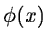which is dilated and translated: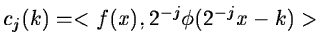(14.9)

Asis a scaling function which has the property:(14.10)

or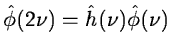(14.11)

where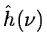is the Fourier transform of the function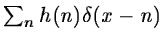. We get:(14.12)

Equation 14.10 permits to compute directly the set cj+1(k) from cj(k). If we start from the set c0(k) we compute all the sets cj(k), with j>0, without directly computing any other scalar product: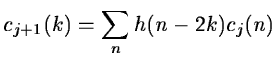(14.13)

At each step, the number of scalar products is divided by 2. Step by step the signal is smoothed and information is lost. The remaining information can be restored using the complementary subspace Wj+1 of Vj+1 in Vj. This subspace can be generated by a suitable wavelet function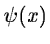with translation and dilation.(14.14)

or(14.15)

We compute the scalar productswith: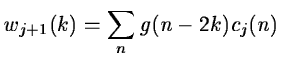(14.16)

With this analysis, we have built the first part of a filter bank . In order to restore the original data, Mallat uses the properties of orthogonal wavelets, but the theory has been generalized to a large class of filters  by introducing two other filtersand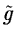named conjugated to h and g. The restoration is performed with:(14.17)

In order to get an exact restoration, two conditions are required for the conjugate filters:

• Dealiasing condition:(14.18)

• Exact restoration: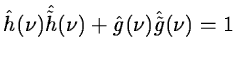(14.19)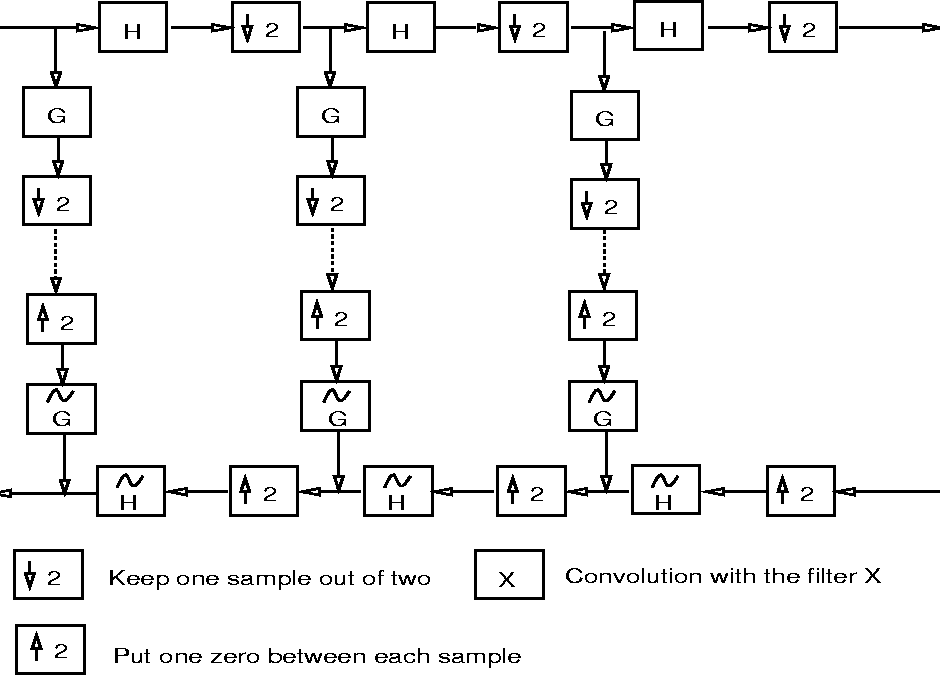In the decomposition, the function is successively convolved with the two filters H (low frequencies) and G (high frequencies). Each resulting function is decimated by suppression of one sample out of two. The high frequency signal is left, and we iterate with the low frequency signal (upper part of figure 14.3). In the reconstruction, we restore the sampling by inserting a 0 between each sample, then we convolve with the conjugate filters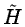and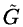, we add the resulting functions and we multiply the result by 2. We iterate up to the smallest scale (lower part of figure 14.3).

Orthogonal wavelets correspond to the restricted case where: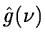=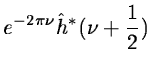(14.20)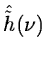=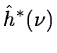(14.21)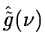=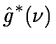(14.22)

and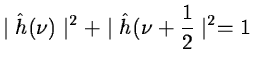(14.23)

We can easily see that this set satisfies the two basic relations 14.18 and 14.19. Daubechies wavelets are the only compact solutions. For biorthogonal wavelets  we have the relations:=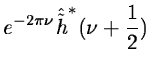(14.24)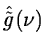=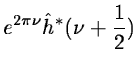(14.25)

and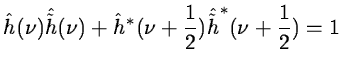(14.26)

We also satisfy relations 14.18 and 14.19. A large class of compact wavelet functions can be derived. Many sets of filters were proposed, especially for coding. It was shown  that the choice of these filters must be guided by the regularity of the scaling and the wavelet functions. The complexity is proportional to N. The algorithm provides a pyramid of N elements.

The 2D algorithm is based on separate variables leading to prioritizing of x and y directions. The scaling function is defined by: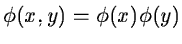(14.27)

The passage from a resolution to the next one is done by: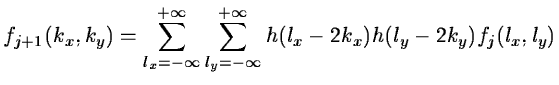(14.28)

The detail signal is obtained from three wavelets:
• a vertical wavelet :• a horizontal wavelet:• a diagonal wavelet: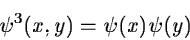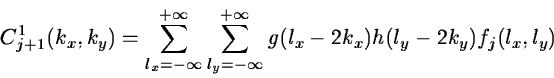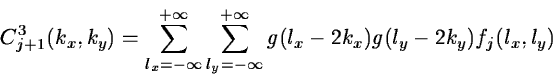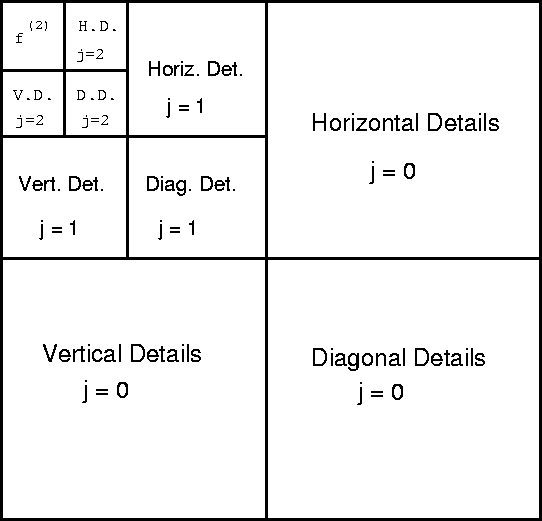The wavelet transform can be interpreted as the decomposition on frequency sets with a spatial orientation.Next: The à trous algorithm Up: The discrete wavelet transform Previous: Introduction
Petra Nass
1999-06-15